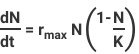### Section 3: Equation Components

#### The “Unused Portion of K”Notice that the logistic equation looks like the exponential equation multiplied by [1-(N/K)]. This mathematical term is what accounts for the density-dependent population growth rate.